Simple Model of Sliding Charge Density Wave

Jiun-Haw Chu May 29, 2007

(Submitted as coursework for Applied Physics 273, Stanford University, Spring 2007)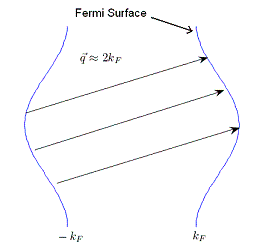Figure 1: The pairing wave vector q nest the quasi 1-d Fermi surface. 

Introduction

The charge density waves are broken symmetry ground states of electrons in metal, which was first proposed by Frohlich in 1954. It was suggested that in an highly anisotropic band structure the instability of Fermi surface will lead to electron-hole pairs condensate. As a result of pairing wave vector the charge density will modulate in a wavelength associated with the Fermi wave vector. This periodic structure have a bandgap near Fermi surface so that it becomes a semiconductor at finite temperature and an insulator at zero temperature. The condensate electrons do not move because they are pinned by impurities and lattice defects. However with large enough electric field applied the whole condensate can slide. 

The symmetry that is broken in charge density waves is translational symmetry. This symmetry breaking makes the center of mass degree of freedom become classical, which enable us to use an simple model to describe the nonlinear transport effects of charge density wave. In this report I shall introduce this model and its predicted transport characteristics compared with experiment. In the end I will discuss the limitation of this model and how we can extend it.

Model of Density Wave Transport

Since the charge density is modulated with a wave vector 2kF, we can model the interaction energy between impurities and collective mode with a periodic potential: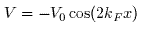where x is the center of mass position of density wave. Because the center of mass degree of freedom becomes classical, it allows us to write a simple equation of motion for x when electric field is applied: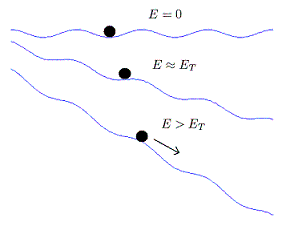Figure 2: The center of mass in a periodic potential under different applied electric field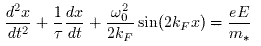This is so-called classical particle model proposed by Gruner.  As shown in Fig. 2, a small electric field will not cause density wave to travel. Instead, there will be a small displacement of center of mass corresponding to a finite polarization. However, when electric field E reaches a threshold value ET, the particle can overcome the potential barrier. ET is given by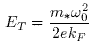To solve the equation of motion, we can neglect the inertial term for small velocities. The original equation then becomes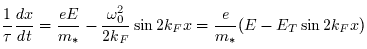We can integrate this equation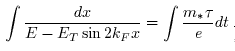and obtain a closed form expression for x(t)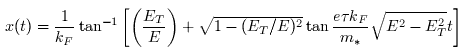and v(t)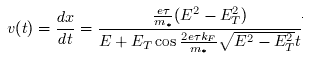Apparently the velocity is a periodic function in time. This implies that when a constant electric field is applied the current will oscillate at a certain frequency. We can also calculated the time averaged value of velocity: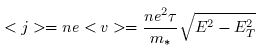The dc conductivity is thus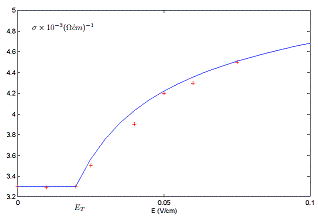Figure 3: The conductivity versus electric field, blue curve is the function given by classical particle model, red crosses are the measured values.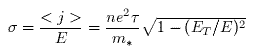Note that it is a strong nonlinear function of the applied dc electric field, and that it has the limiting value ne2&tau/m* when the applied field is large compared to the threshold value. This nonlinear behavior was first observed in NbSe3 in 1976 by Monceau et al.. The experimental values are compared with the model in Fig. 3. The experiment was performed at T = 49K. (NbSe3 has charge density wave transitions at T = 129K and 49K.) Note that there is still ohmic conductivity for E below ET. When temperature is nonzero, thermally excited electrons also contribute to conduction.

Limitation of the Model

In the classical particle model we describe the density wave motion solely by its center of mass position. We treate it as a rigid condensate and disregard the internal deformation caused by the impurity. This is valid only when sample size is smaller than the phase-phase correlation length - which is of the orders of microns for pure specimens. A more realistic equation of motion, which considers the local distortion of density wave phase, is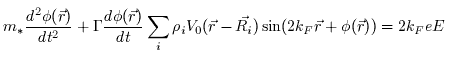where Ri, the position of impurities, is a random number. Numerical simulation has been applied to study the complex nonlinear dynamics of this model. A different approach treats the problem as a critical phenomenon and attempts to calculate the critical exponent of the electric field dependence for E near ET. Nevertheless, the classical particle model gives us a correct qualitative understanding of how a charge density wave slides in the presence of a sufficiently large electric field.

© 2007 Jiun-Haw Chu. The author grants permission to copy, distribute and display this work in unaltered form, with attribution to the author, for noncommercial purposes only. All other rights, including commercial rights, are reserved to the author.

References

 H. Frohlich, "On the Theory of Superconductivity: The One-Dimensional Case," Proc. Roy. Soc. London, Ser. A 223, 296 (1954).

 G. Gruner, Density Waves in Solids (Addison-Wesley, 1994).

 G. Gruner, A. Zawadowski, and P. M. Chaikin, "Nonlinear Conductivity and Noise due to Charge-Density-Wave Depinning in NbSe3," Phys. Rev. Lett. 46, 511 (1981).

 P. Monceau, N. P. Ong, and A. M. Portis, A. Meerschaut and J. Rouxel, "Electric Field Breakdown of Charge-Density-Wave Induced Anomalies in NbSe3 ," Phys. Rev. Lett. 37, 602 (1976).

 O. Narayan and D. S. Fisher, "Critical behavior of sliding charge-density waves in 4-dimensions," Phys. Rev. B 46, 11520 (1992).